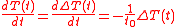xConvective heat transferEncyclopedia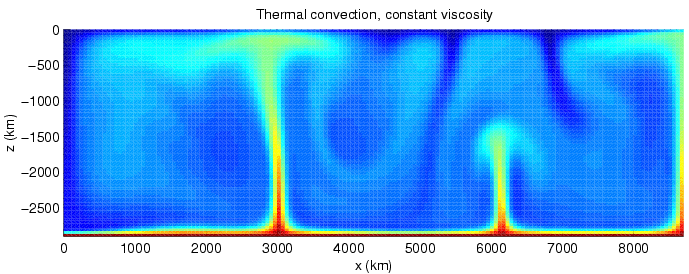Convective heat transfer, often referred to as convection, is the transfer of heat from one place to another by the movement of fluids. The presence of bulk motion of the fluid enhances the heat transfer between the solid surface and the fluid. Convection is usually the dominant form of heat transfer in liquids and gases. Although often discussed as a third method of heat transfer, convective heat transfer actually describes the combined effects of conduction (heat diffusion), plus heat transfer by bulk fluid flow, a process technically termed "heat advection
Advection, in chemistry, engineering and earth sciences, is a transport mechanism of a substance, or a conserved property, by a fluid, due to the fluid's bulk motion in a particular direction. An example of advection is the transport of pollutants or silt in a river. The motion of the water carries...

."

The term convection
Convection
Convection is the movement of molecules within fluids and rheids. It cannot take place in solids, since neither bulk current flows nor significant diffusion can take place in solids....

can refer to transfer of heat with any fluid movement, but advection
Advection, in chemistry, engineering and earth sciences, is a transport mechanism of a substance, or a conserved property, by a fluid, due to the fluid's bulk motion in a particular direction. An example of advection is the transport of pollutants or silt in a river. The motion of the water carries...

is the more precise term for the transfer due only to bulk fluid flow. The process of transfer of heat from a solid to a fluid, or the reverse, requires not only transfer of heat by bulk motion of the fluid, but also diffusion/conduction of heat through the still boundary layer next to the solid. Thus, this process with a moving fluid requires both diffusion and advection of heat, a summed process that is generally called convection.

Convection can be "forced" by movement of a fluid by means other than buoyancy forces (for example, a water pump in an automobile engine). In some cases, natural buoyancy forces alone are entirely responsible for fluid motion when the fluid is heated, and this process is called "natural convection." An example is the draft in a chimney or around any fire. In natural convection, an increase in temperature produces a reduction in density, which causes fluid motion due to pressures and forces when fluids of different densities are affected by gravity (or any g-force). For example, when water is heated on a stove
Stove
A stove is an enclosed heated space. The term is commonly taken to mean an enclosed space in which fuel is burned to provide heating, either to heat the space in which the stove is situated or to heat the stove itself, and items placed on it...

, hot water from the bottom of the pan rises, displacing the colder denser liquid which falls. After heating has stopped, mixing and conduction from this natural convection eventually result in a nearly homogeneous density, and even temperature.

The convection heat transfer mode is comprised to two mechanisms. In addition to energy transfer due to random molecular motion (diffusion), energy is also transferred by bulk, or macroscopic, motion of the fluid. This motion is associated with the fact that, at any instant, large numbers of molecules are moving collectively or as aggregates. Such motion, in the presence of a temperature gradient, contributes to heat transfer. Because the molecules in aggregate retain their random motion, the total heat transfer is then due to the superposition of energy transport by random motion of the molecules and by the bulk motion of the fluid. It is customary to use the term convection when referring to this cumulative transport and the term advection when referring to the transport due to bulk fluid motion.

## OverviewConvection is the transfer of heat from one place to another by the movement of fluids. The presence of bulk motion of the fluid enhances the heat transfer between the solid surface and the fluid. Convection is usually the dominant form of heat transfer in liquids and gases. Although often discussed as a third method of heat transfer, convection actually describes the combined effects of conduction and fluid flow.

There are two types of convective heat transfer
• Free or natural convection
Natural convection
Natural convection is a mechanism, or type of heat transport, in which the fluid motion is not generated by any external source but only by density differences in the fluid occurring due to temperature gradients. In natural convection, fluid surrounding a heat source receives heat, becomes less...

: when the fluid motion is caused by buoyancy forces that result from the density variations due to variations of temperature in the fluid. In the absence of an external source, when the mass of the fluid is in contact with a hot surface, its molecules separate and scatter, causing the mass of fluid to become less dense. When this happens, the fluid is displaced vertically or horizontally while the cooler fluid gets denser and the fluid sinks. Thus the hotter volume transfers heat towards the cooler volume of that fluid. Familiar examples are the upward flow of air due to a fire or hot object and the circulation of water in a pot that is heated from below.

• Forced convection
Forced convection
Forced convection is a mechanism, or type of heat transport in which fluid motion is generated by an external source...

: when the fluid is forced to flow over the surface by external source such as fans, stirrers, and pumps, creating an artificially induced convection current.

Internal and external flow can also classify convection. Internal flow occurs when the fluid is enclosed by a solid boundary such as a flow through a pipe. An external flow occurs when the fluid extends indefinitely without encountering a solid surface. Both of these convections, either natural or forced, can be internal or external because they are independent of each other. The bulk temperature
Bulk temperature
In fluid dynamics, the bulk temperature, or the average fluid bulk temperature, is a convenient reference point for evaluating properties related to convective heat transfer, particularly in applications related to flow in pipes and ducts....

, or the average fluid temperature, is a convenient reference point for evaluating properties related to convective heat transfer, particularly in applications related to flow in pipes and ducts.

For a visual experience of natural convection, a glass that is full of hot water filled with red food dye may be placed inside a fish tank with cold, clear water. The convection currents of the red liquid will be seen to rise and also fall, then eventually settle, illustrating the process as heat gradients are dissipated.

## Equations (Newton's law of cooling)

Convection-cooling can sometimes be described by Newton's law of cooling in cases where the heat transfer coefficient is independent or relatively independent of the temperature difference between object and environment. This is sometimes true, but is not guaranteed to be the case (see other situations below where the transfer coefficient is temperature dependent).

Newton's law, which requires a constant heat transfer coefficient, states that the rate of heat loss of a body is proportional to the difference in temperatures between the body and its surroundings. The rate of heat transfer in such circumstances is derived below:

Newton's cooling law is a solution of the differential equation given by Fourier's law: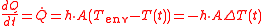where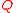is the thermal energy in joule
Joule
The joule ; symbol J) is a derived unit of energy or work in the International System of Units. It is equal to the energy expended in applying a force of one newton through a distance of one metre , or in passing an electric current of one ampere through a resistance of one ohm for one second...

s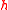is the Heat transfer coefficient (assumed independent of T here)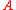is the surface area of the heat being transferred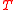is the temperature of the object's surface and interior (since these are the same in this approximation)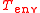is the temperature of the environment; i.e. the temperature suitably far from the surface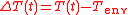is the time-dependent thermal gradient between environment and object

The heat transfer coefficient h depends upon physical properties of the fluid and the physical situation in which convection occurs. Therefore, a single usable heat transfer coefficient (one that does not vary significantly across the temperature-difference ranges covered during cooling and heating) must be derived or found experimentally for every system analyzed. Formulas and correlations are available in many references to calculate heat transfer coefficients for typical configurations and fluids. For laminar flows, the heat transfer coefficient is rather low compared to the turbulent flows; this is due to turbulent flows having a thinner stagnant fluid film layer on heat transfer surface. However, note that Newton's law breaks down if the flows should transition between laminar or turbulent flow, since this will change the heat transfer coefficient h which is assumed constant in solving the equation.

Newton's law requires that internal heat conduction within the object be large in comparison to the loss/gain of heat by convection (lumped capacitance model
Lumped capacitance model
A lumped capacitance model, also called lumped system analysis, reduces a thermal system to a number of discrete “lumps” and assumes that the temperature difference inside each lump is negligible. This approximation is useful to simplify otherwise complex differential heat equations...

), and this may not be true (see heat transfer
Heat transfer
Heat transfer is a discipline of thermal engineering that concerns the exchange of thermal energy from one physical system to another. Heat transfer is classified into various mechanisms, such as heat conduction, convection, thermal radiation, and phase-change transfer...

). Also, an accurate formulation for temperatures may require analysis based on changing heat transfer coefficients at different temperatures, a situation frequently found in free-convection situations, and which precludes accurate use of Newton's law. Assuming these are not problems, then the solution can be given if heat transfer within the object is considered to be far more rapid than heat transfer at the boundary (so that there are small thermal gradients within the object). This condition, in turn, allows the heat in the object to be expressed as a simple product of the object's mass, its heat capacity, and its temperature, as in the following section:

### Solution in terms of object heat capacity

If the entire body is treated as lumped capacitance thermal energy reservoir, with a total thermal energy content which is proportional to simple total heat capacity
Heat capacity
Heat capacity , or thermal capacity, is the measurable physical quantity that characterizes the amount of heat required to change a substance's temperature by a given amount...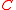, and, the temperature of the body, or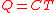. It is expected that the system will experience exponential decay with time in the temperature of a body.

From the definition of heat capacitycomes the relation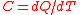. Differentiating this equation with regard to time gives the identity (valid so long as temperatures in the object are uniform at any given time):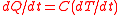. This expression may be used to replace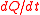in the first equation which begins this section, above. Then, if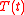is the temperature of such a body at time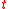, and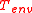is the temperature of the environment around the body: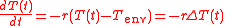where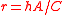is a positive constant characteristic of the system, which must be in units of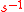, and is therefore sometimes expressed in terms of a characteristic time constant
Time constant
In physics and engineering, the time constant, usually denoted by the Greek letter \tau , is the risetime characterizing the response to a time-varying input of a first-order, linear time-invariant system.Concretely, a first-order LTI system is a system that can be modeled by a single first order...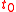given by: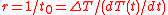. Thus, in thermal systems,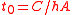. (The total heat capacity
Heat capacity
Heat capacity , or thermal capacity, is the measurable physical quantity that characterizes the amount of heat required to change a substance's temperature by a given amount...of a system may be further represented by its mass-specific heat capacity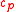multiplied by its mass, so that the time constantis also given by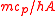).

The solution of this differential equation, by standard methods of integration and substitution of boundary conditions, gives: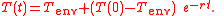If: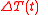is defined as :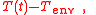where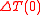is the initial temperature difference at time 0,

then the Newtonian solution is written as: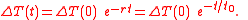This same solution is more immediately apparent if the initial differential equation is written in terms of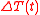, as a single function of time to be found, or "solved for."
'